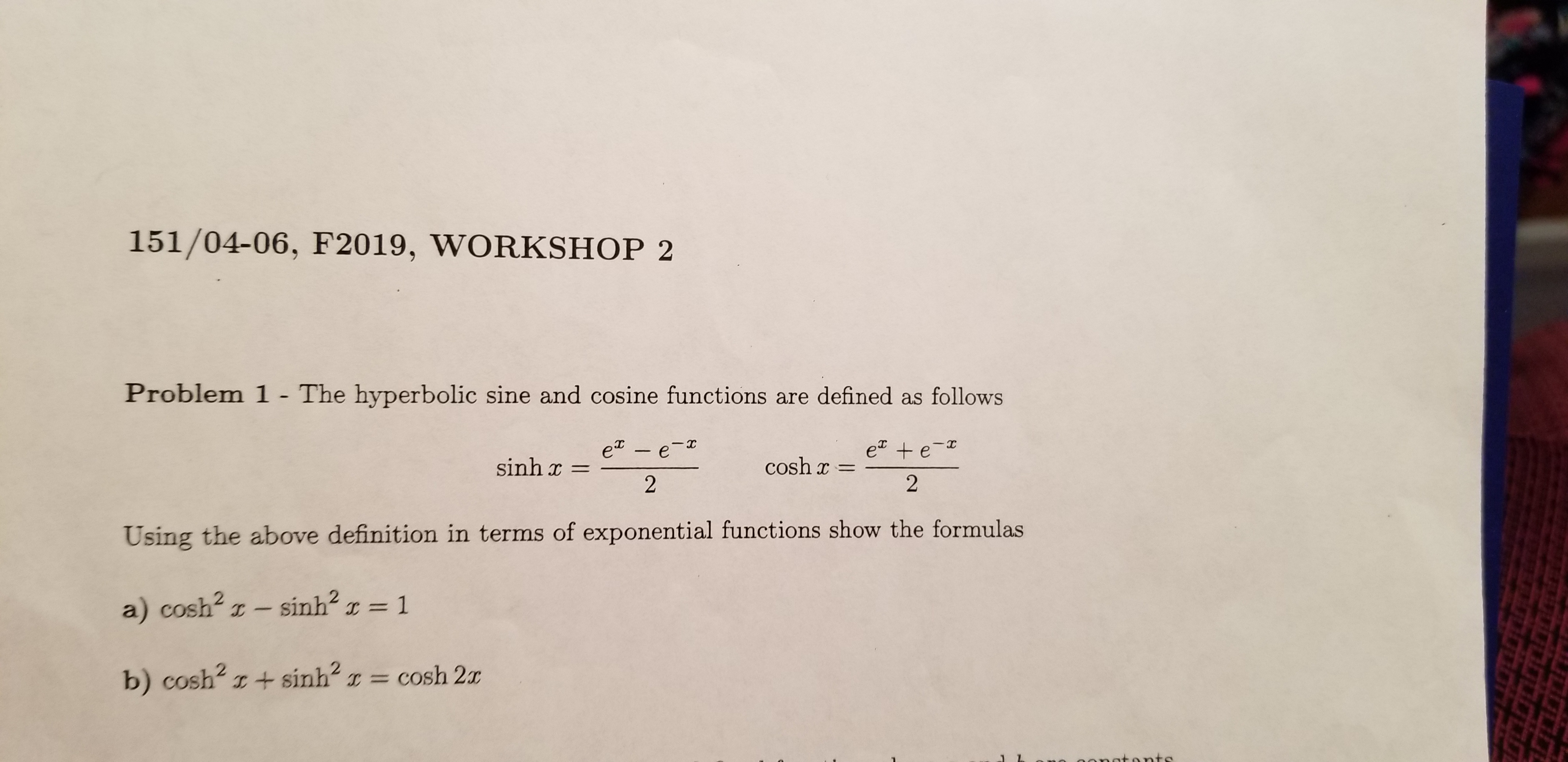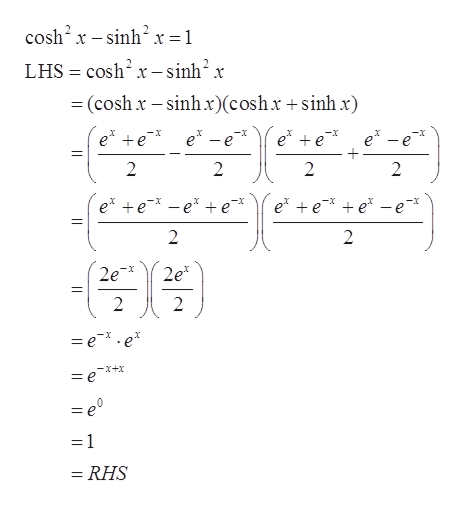# 151/04-06, F2019, WORKSHOP 2Problem 1 - The hyperbolic sine and cosine functions are defined as followse + e-2e - e-sinh xcosh2Using the above definition in terms of exponential functions show the formulasa) cosh2 z- sinh21C =ICCO= cosh 2xb) cosh2 I+sinhtonts

Question
11 views

Problem 1 in attached imagehelp_outlineImage Transcriptionclose151/04-06, F2019, WORKSHOP 2 Problem 1 - The hyperbolic sine and cosine functions are defined as follows e + e- 2 e - e- sinh x cosh 2 Using the above definition in terms of exponential functions show the formulas a) cosh2 z- sinh2 1 C = IC CO = cosh 2x b) cosh2 I+sinh tonts fullscreen
check_circle

Step 1

Given,

Step 2

Part (a): To show

...help_outlineImage Transcriptionclosecosh2 x-sinh2x =1 LHS cosh2 x-sinh2 x (cosh x-sinhx)(coshx +sinh x) X -x e -e e e e -e e +e 2 2 2 2 ex e-e e e e e e 2 2 2e 2e* 2 2 = e.e* -x+x = e = e° =1 = RHS fullscreen

### Want to see the full answer?

See Solution

#### Want to see this answer and more?

Solutions are written by subject experts who are available 24/7. Questions are typically answered within 1 hour.*

See Solution
*Response times may vary by subject and question.
Tagged in

### Other